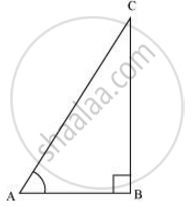Share

# State whether the following are true or false. Justify your answer. The value of tan A is always less than 1. - Mathematics

Course

#### Question

The value of tan A is always less than 1.

#### Solution

Consider a ΔABC, right-angled at B.tan A = ("Side opposite to"angle A)/("Side adjacent to"angleA)

= 12/5

But 12/5 > 1

∴tan A > 1

So, tan A < 1 is not always true.

Hence, the given statement is false.

Is there an error in this question or solution?

#### APPEARS IN

NCERT Solution for Mathematics Textbook for Class 10 (2019 (Latest))
Chapter 8: Introduction to Trigonometry
Ex. 8.1 | Q: 11.1 | Page no. 181
RD Sharma Solution for Class 10 Maths (2018 (Latest))
Chapter 10: Trigonometric Ratios
Ex. 10.1 | Q: 36.1 | Page no. 26
RD Sharma Solution for Class 10 Maths (2018 (Latest))
Chapter 10: Trigonometric Ratios
Ex. 10.1 | Q: 36.1 | Page no. 26